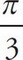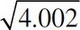# AP Calculus AB Practice Test 25

### Test Information7 questions21 minutes

Calculator Allowed

1. Approximate the area under the curve y = x2 + 2 from x = 1 to x = 2 using four midpoint rectangles.

2. Find the area under the curve y = x2 + 2 from x = 1 to x = 2.

3. The side of a cube is increasing at a rate of 3 inches per second. At the instant when the side of the cube is 6 inches long. What is the rate of change (in inches/second) of the surface area of the cube?

4. If the position of a particle is given by x(t) = 3t3 - 2t2 - 16 where t > 0. When does the particle change direction?

5. The radius of a sphere is increased from 9 cm to 9.05 cm. Estimate the change in volume.

6. Find an equation of the line tangent to the curve represented by x = 4cost + 2 and y = 2sint at t =.

7. Use differentials to approximate.